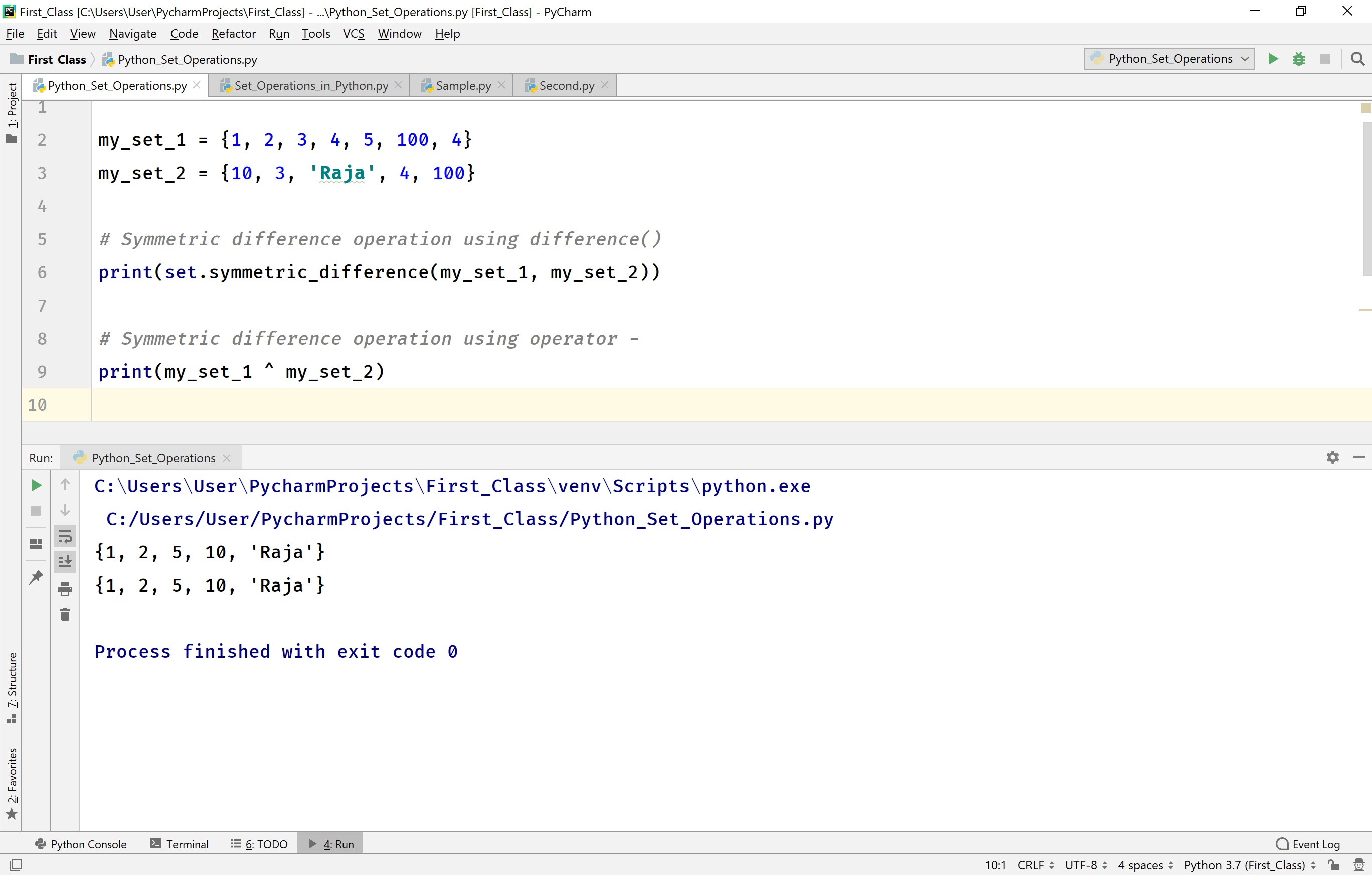The perfect place for easy learning...

×

# Python Sets

In Python, a set is a collection of unordered and unindexed elements of different data types. That means sets are similar to the lists and tuples. But, the elements of a set are maintained without any index and random order. The elements are immutable however set itself is mutable. That means the elements of a set can not be modified whereas the entire set can be modified or redefined. In Python, the set data type (data structure) has implemented with a class known as a set. All the elements of a set must be enclosed in curly braces, and each element must be separated with a comma symbol.

## Creating a set in Python

The general syntax for creating a set in Python is as follows.

### Syntax

`set_name = {element_1, element_2, element_3, ...}`

For example, consider the following code for creating a set which stores the details of a student.

# Python code to illustrate creating a set

``````
student_data = {1, 'Rama', '2nd Year', 'CSE', 85.80}
print(type(student_data))
print(student_data)
```
```

When we run the above code, it produces the output as follows.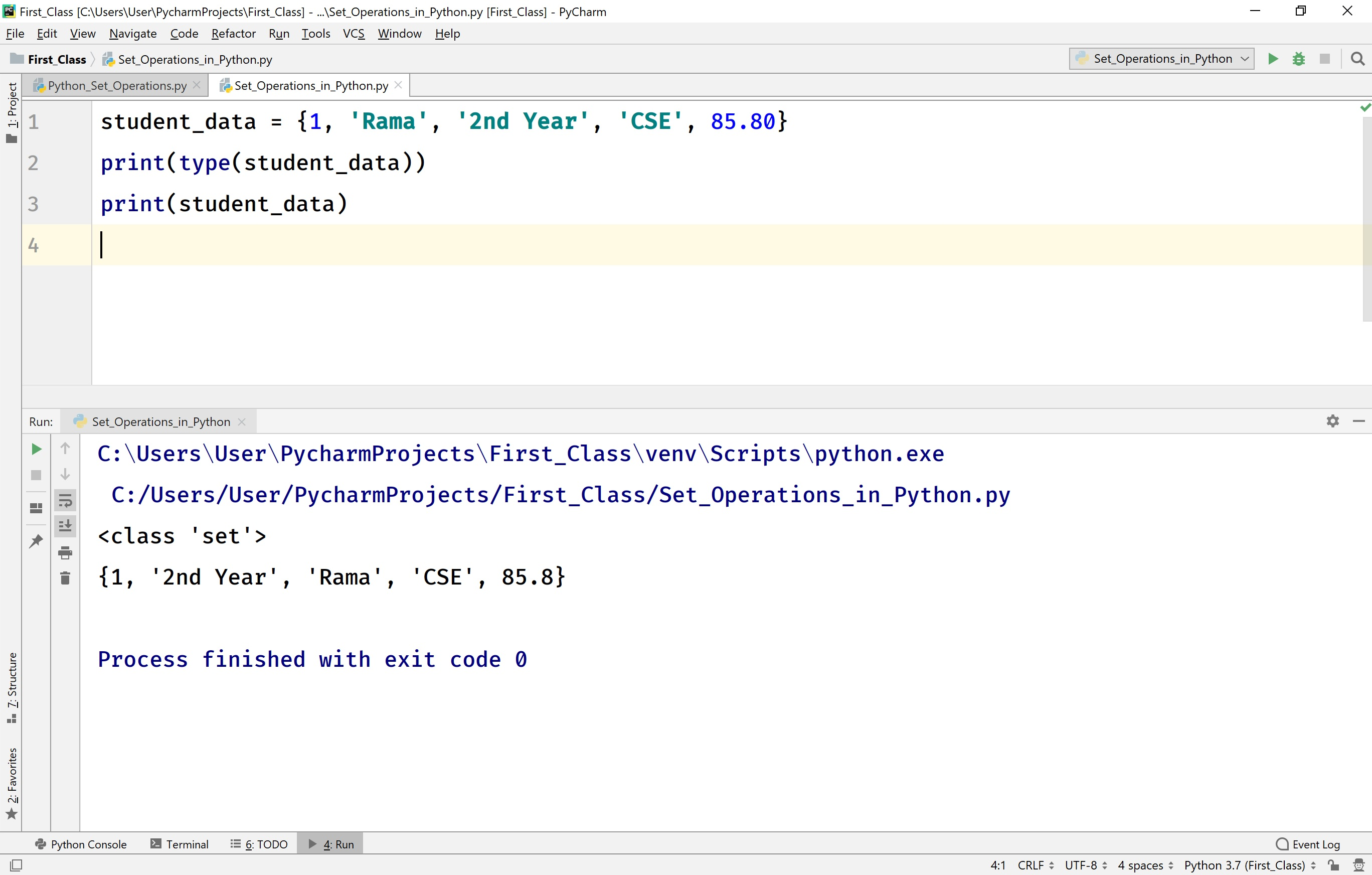In Python, a set can also be created using the set( ) constructor. The set() constructor takes only one argument.

### Syntax

`set_name = set((element_1, element_2, element_3, ...))`

For example, consider the following code for creating a set using set() constructor which stores the details of a college.

# Python code to illustrate creating a set using set() constructor.

``````
college_info = set(('JNTUH', 'MRIT', 'MLTM'))
print(type(college_info))
print(college_info)
```
```

When we run the above code, it produces the output as follows.## Accessing Elements of a set in Python

In Python, the set elements are organized without any index values. So, accessing individual elements of a set is not allowed. However, we can access the entire set using the name of the set.

For example, consider the following code for accessing the entire set using the name of the set.

# Python code to illustrate accessing the entire set.

``````
my_set = {1, 50, 'raja', 100.99, 'Sam'}
print(type(my_set))
print(my_set)
```
```

When we run the above code, it produces the output as follows.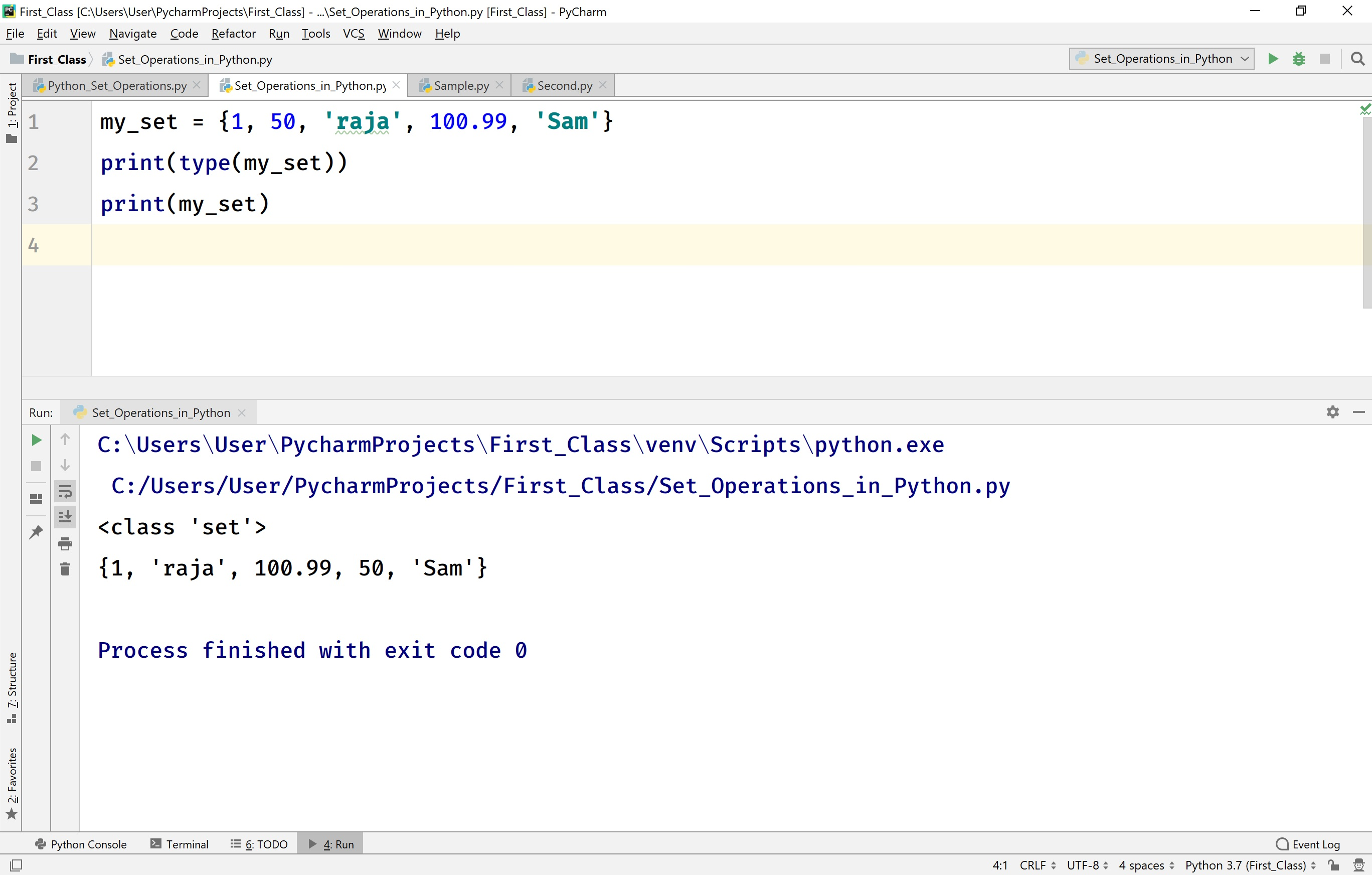## Changing an Element of a set in Python

In Python, the elements of a set are immutable. So, modification of individual elements in a set is not allowed.

• In Python, the whole set is mutable. So, we can modify or redefine the entire set.

## Looping through a set in Python

In Python, we can loop through a set using for statement with a membership operator in.

For example, consider the following code to loop through a set.

# Python code to illustrate loop through a set.

``````
student_data = {1, 'Rama', '2nd Year', 'CSE', 85.80}
for element in student_data:
print(f'Element from the set is - {element}')
```
```

When we run the above code, it produces the output as follows.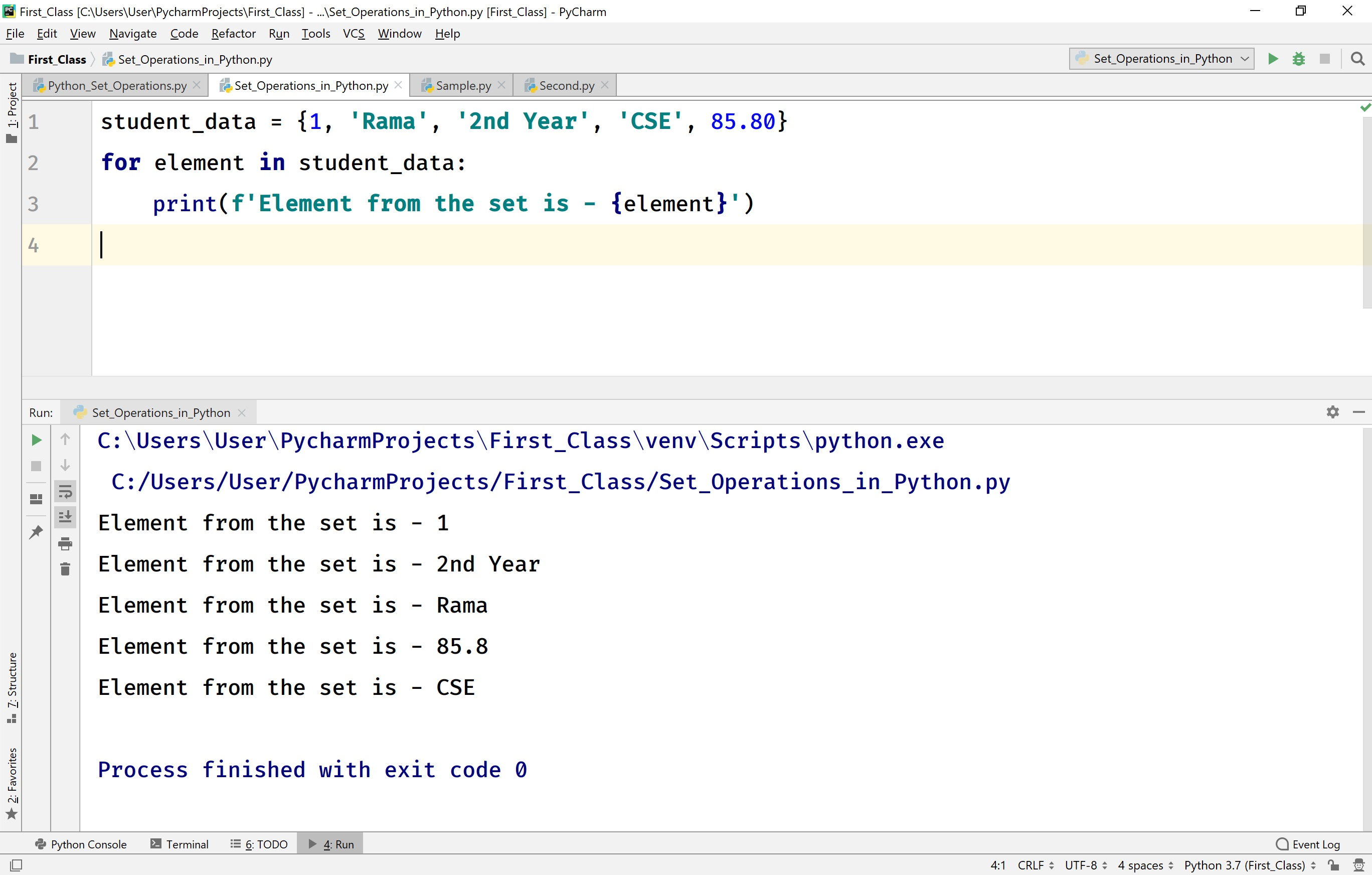## Existence of an element in a set in Python

In Python, we can test whether an element is present in a set or not using a membership operator 'in'.

For example, consider the following code to test the existence of an element in a set.

# Python code to illustrate the existence of an element in a set.

``````
student_data = {1, 'Rama', '2nd Year', 'CSE', 85.80}
if 'CSE' in student_data:
print(f'CSE is found in the set {student_data}!!!')
else:
print(f'CSE is not found in the set {student_data}!!!')
```
```

When we run the above code, it produces the output as follows.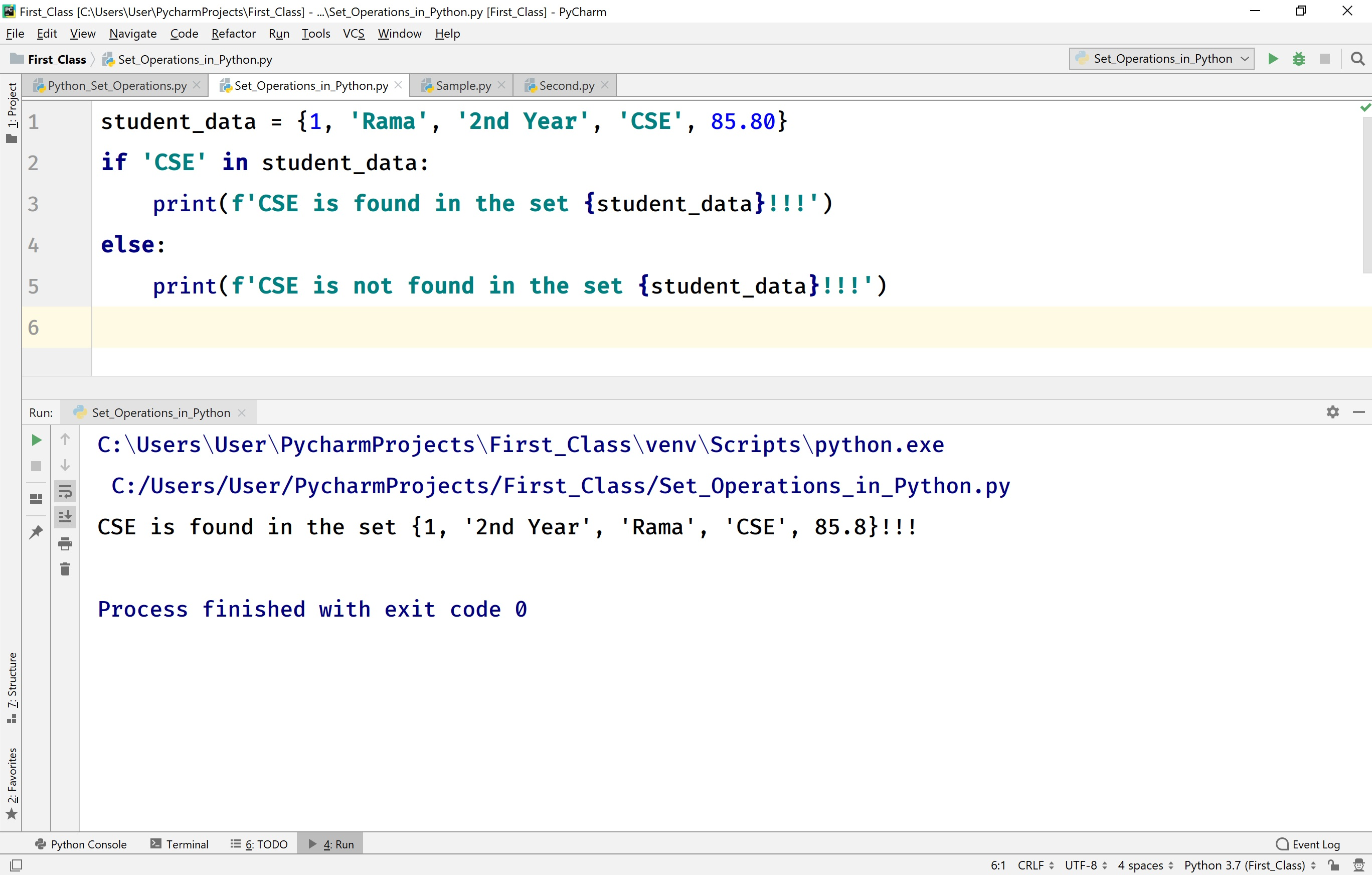## Finding the length of a set in Python

The Python provides a built-in function len( ) to find the length of a set. Here, the length of a set is the total number of elements in that set.

For example, consider the following code to find the length of a set.

# Python code to illustrate the length of a set.

``````
student_data = {1, 'Rama', '2nd Year', 'CSE', 85.80}
tuple_length = len(student_data)
print(f'Length of the tuple {student_data} is {tuple_length}')
```
```

When we run the above code, it produces the output as follows.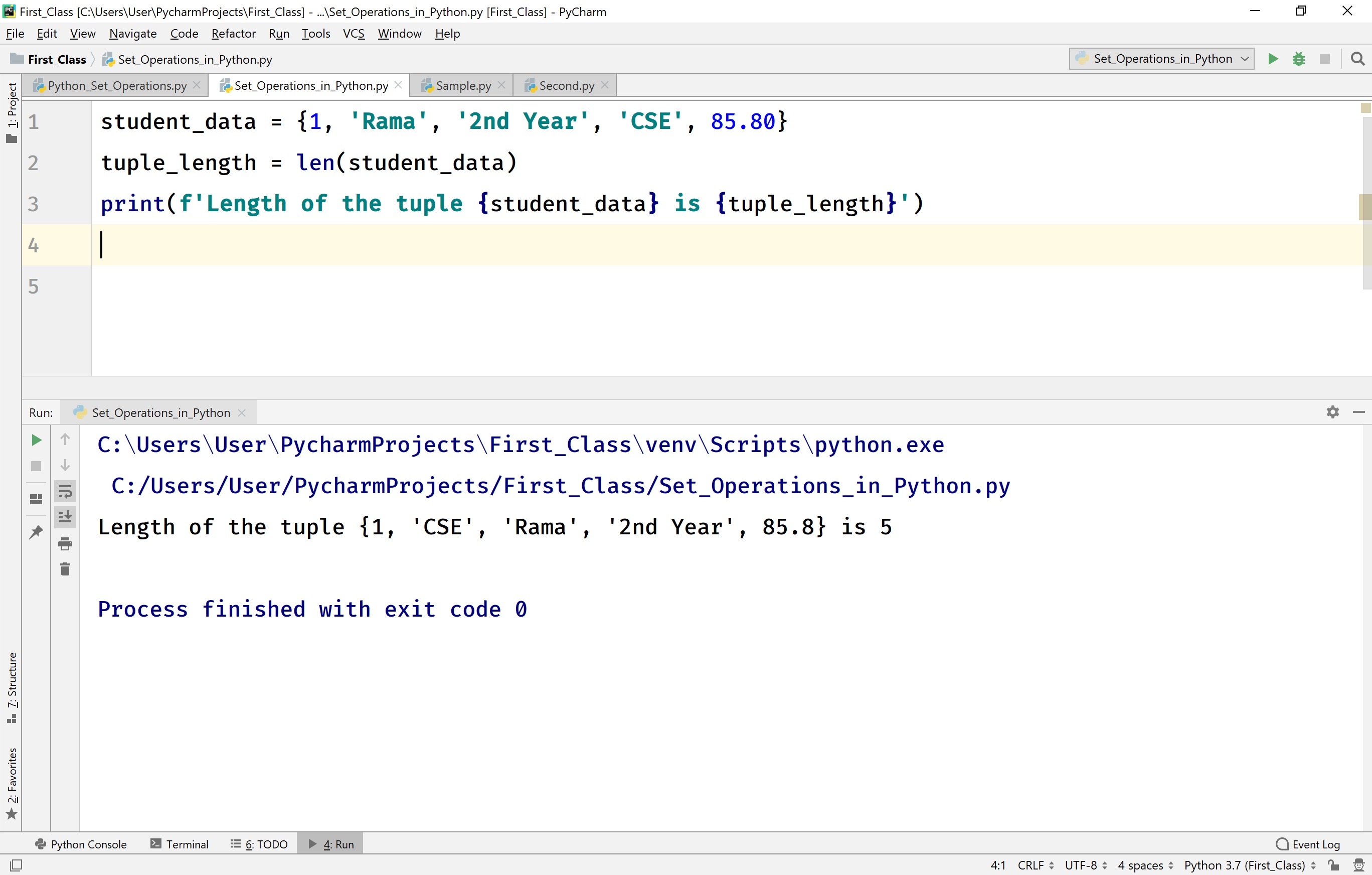## Adding an element to the existing set in Python

Adding elements to the existing set in Python is performed using the following built-in methods.

• add( value ) - This method adds the given element to the existing set.

• update( list_of_values ) - This method adds a given list of elements to the existing set.

For example, consider the following code.

# Python code to illustrate adding an element to a set.

``````
my_set = {1, 2, 3, 4, 5, 6, 7, 8, 9}
print(f'The set is - {my_set}')

print(f'The set after adding 10 is - {my_set}')

# Adding multiple elements using update() method
my_set.update([100, 200])
print(f'The set after adding 100 and 200 is - {my_set}')
```
```

When we run the above code, it produces the output as follows.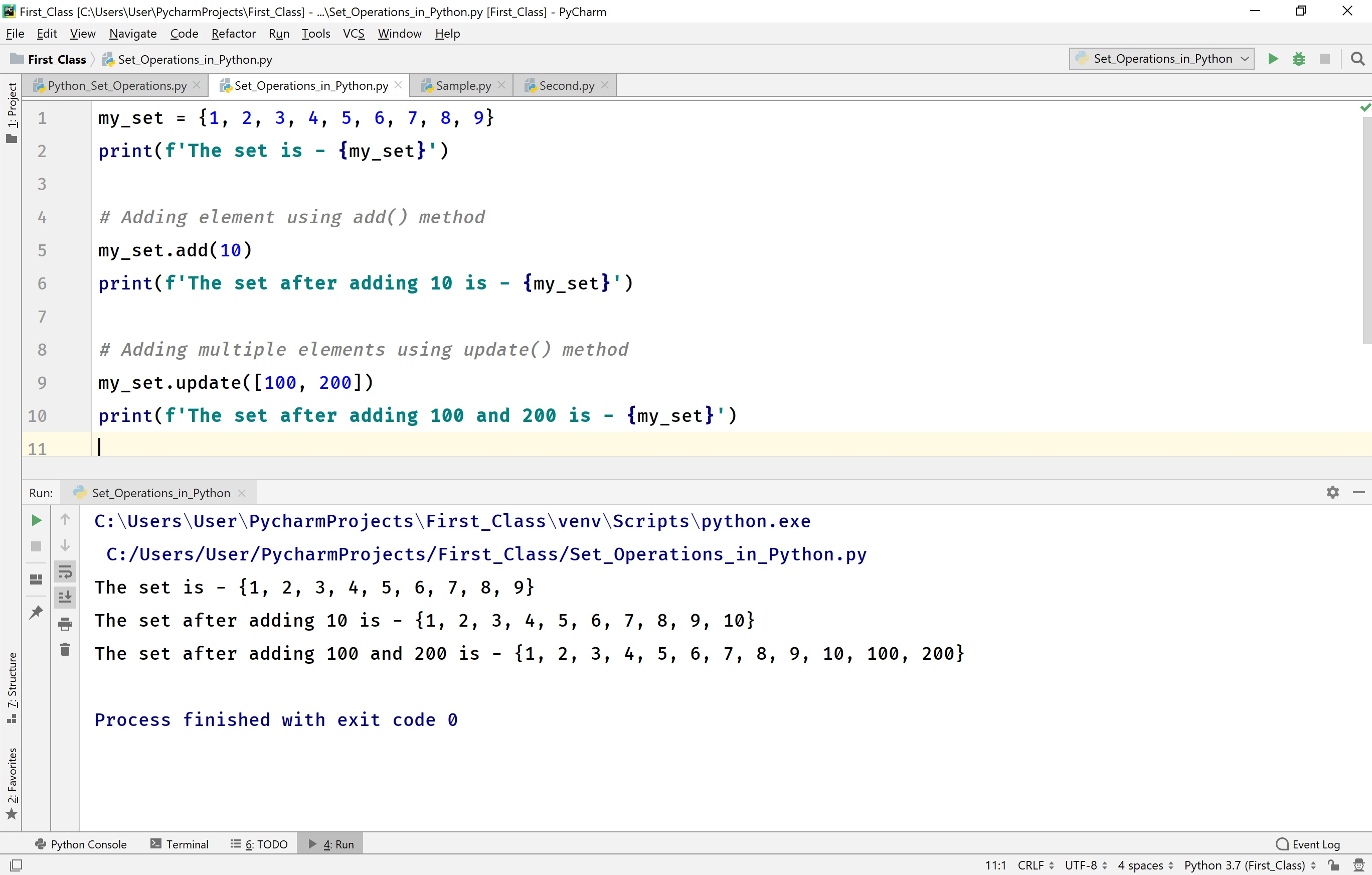## Removing elements from a set in Python

In Python, removing an element from the existing tuple is performed using the following built-in methods.

• discard( value ) - This method removes the given element from the set. This method returns a None value. When the given element is not found in the set, then it simply ignores it but does not cause any error.

• remove( value ) - This method removes the given element from the set. This method returns a None value. When the given element is not found in the set, then it causes an error.

• pop( ) - This method removes the last element from the set. This method returns the removed value. As the set is an unordered sequence of elements, so we will not know what element that gets removed.

• clear( ) - This method removes all the elements from the set. That means the clear( ) method make the set empty. This method returns the None value.

• del keyword - This keyword deletes the set completely. Once the del keyword has used on a set, we can not access it in the rest of the code.

For example, consider the following code.

# Python code to illustrate remove operations in a set.

``````
my_set = {1, 2, 3, 4, 5, 6, 7, 8, 9, 10}
print(f'The set is - {my_set}')
print(f'The set after discarding 5 is {my_set}')
my_set.remove(3)
# my_set.remove(30) # Generates an error
print(f'The set after removing 3 is {my_set}')
my_set.pop()
print(f'The set after pop is {my_set}')
my_set.clear()
print(f'The set after clear is {my_set}')
del my_set
# print(f'The set after del is {my_set}') # Generates an error
```
```

When we run the above code, it produces the output as follows.## Finding Maximum and Minimum value in a tuple

The Python programming language provides built-in methods max( ) and min( ) to find the maximum and minimum elements in a set.

For example, consider the following code.

# Python code to illustrate max( ) and mim( ) methods in a set.

``````
my_set = {1, 2, 3, 4, 5, 6, 7, 8, 9, 10, 4}
print(f'The set is - {my_set}')
print(f'The maximum element is - {max(my_set)}')
print(f'The minimum element is - {min(my_set)}')
```
```

When we run the above code, it produces the output as follows.## Sorting the elements of a set in Python

The Python provides a built-in method sorted( ) to sort all the elements of a set. The method sorted( ) returns a list of the element in sorted order. The sorted( ) method arranges the set elements in increasing order by default. To sort the elements in decreasing order, we need to pass an argument reverse = True to sorted( ) method.

For example, consider the following code.

# Python code to illustrate sort( ) in a set.

``````
my_set = {1, 2, 3, 42, 50, 6, 7, 8, 9, 10, 4}
print(f'The set is - {my_set}')
my_set = sorted(my_set)
print(f'The sorted elements (increasing order) of set is {my_set}')
my_set = sorted(my_set, reverse=True)
print(f'The sorted elements (decreasing order) of set is {my_set}')
```
```

When we run the above code, it produces the output as follows.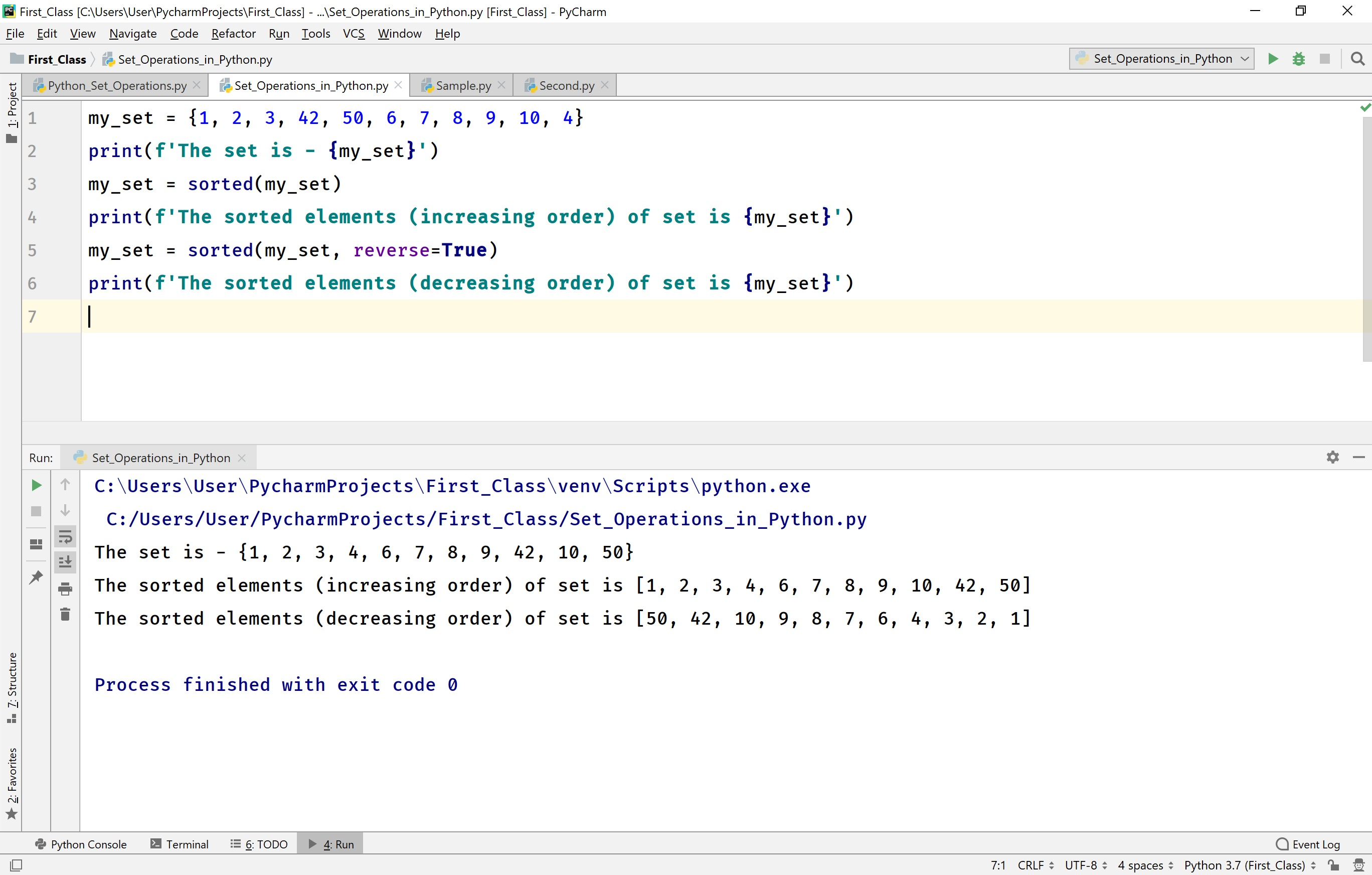• In Python, the functions max( ), min( ), and sorted( ) can be used with the set only if the set contains all elements of numerical type.

## Union of sets in Python

The Python provides the following ways to perform union of two or more number of sets.

• union( ) - union( ) is a built-in method used to perform a union of two or more number of sets.

• Operator '|' - This operator is used to perform a union of two or more number of sets.

For example, consider the following code.

# Python code to illustrate the union of sets.

``````
my_set_1 = {1, 2, 3, 4, 5, 100, 4}
my_set_2 = {10, 3, 'Raja', 4, 100}
my_set_3 = {1000, 3, 'Rama', 14, 10000, 5}
# Union operation using method union()
print(my_set_1.union(my_set_2, my_set_3))

# Union operation using operator |
print(my_set_1 | my_set_2 | my_set_3)
```
```

When we run the above code, it produces the output as follows.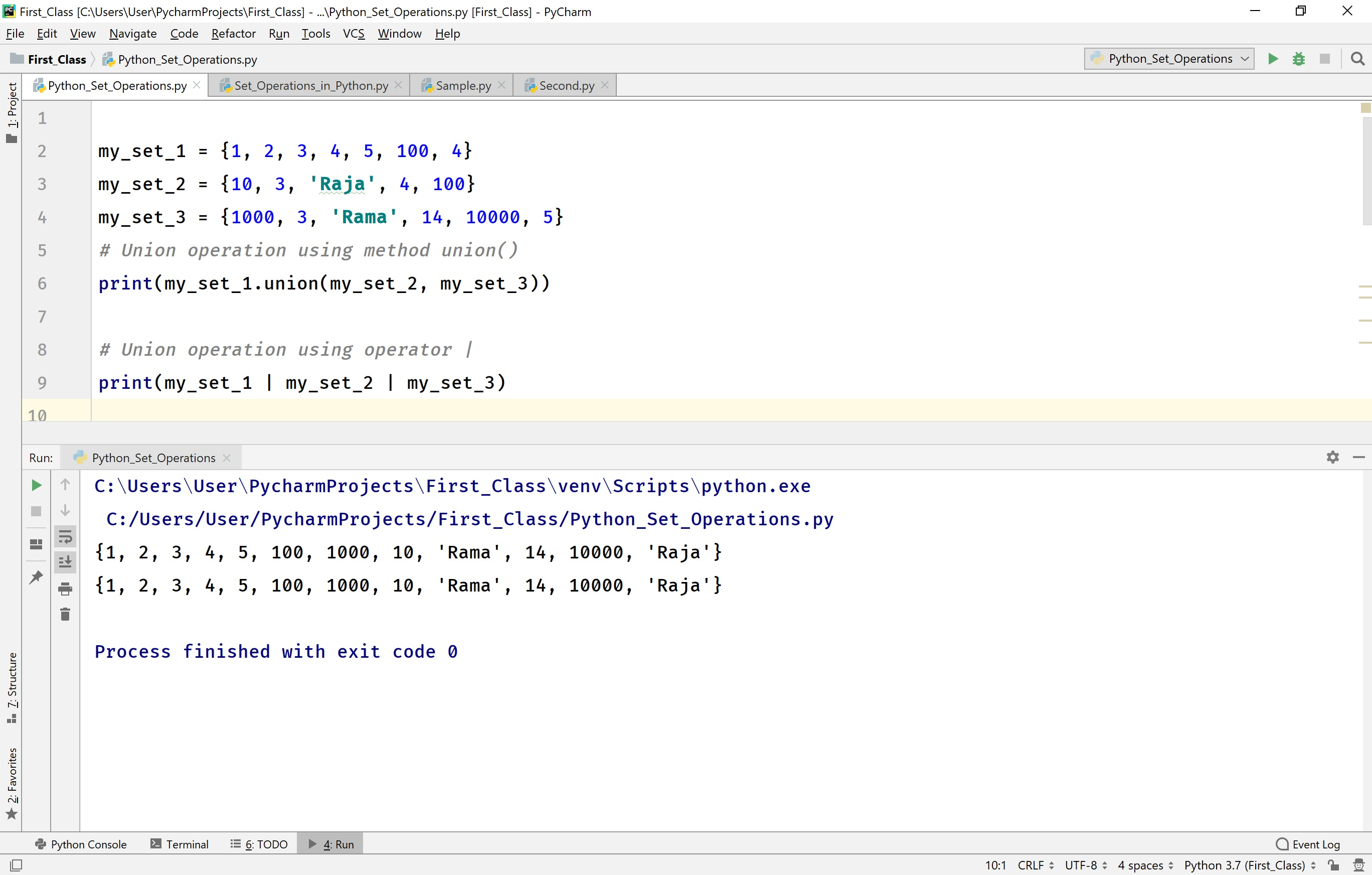## The intersection of sets in Python

The Python provides the following ways to perform an intersection of two or more number of sets.

• intersection( ) - intersection( ) is a built-in method used to perform intersection of two or more number of sets.

• Operator '&' - This operator is used to perform the intersection of two or more number of sets.

For example, consider the following code.

# Python code to illustrate the intersection of sets.

``````
my_set_1 = {1, 2, 3, 4, 5, 100, 4}
my_set_2 = {10, 3, 'Raja', 4, 100}

# Intersection using method intersection()
print(set.intersection(my_set_1, my_set_2))

# Intersection using operator &
print(my_set_1 & my_set_2)
```
```

When we run the above code, it produces the output as follows.## The difference of sets in Python

The Python provides the following ways to perform the difference operation of two or more number of sets. The difference operation on sets is the results with a set which contains the elements from the first operand set those are not in the second operand set.

• difference( ) - difference( ) is a built-in method used to perform difference of two or more number of sets.

• Operator '-' - This operator is used to perform a difference of two or more number of sets.

For example, consider the following code.

# Python code to illustrate the difference of sets.

``````
my_set_1 = {1, 2, 3, 4, 5, 100, 4}
my_set_2 = {10, 3, 'Raja', 4, 100}

# Difference operation using difference()
print(set.difference(my_set_1, my_set_2))

# Difference operation using operator -
print(my_set_1 - my_set_2)
```
```

When we run the above code, it produces the output as follows.## Symmetric difference of sets in Python

The Python provides the following ways to perform the symmetric difference operation of two or more number of sets. The symmetric difference operation on sets is the results with a set which contains the elements from all the operand sets but the common elements from all the sets are not included.

• symmetric_difference( ) - The symmetric_difference( ) is a built-in method used to perform symmetric difference operation of two or more number of sets.

• Operator '^' - This operator is used to perform a symmetric difference of two or more number of sets.

For example, consider the following code.

# Python code to illustrate the symmetric difference of sets.

``````
my_set_1 = {1, 2, 3, 4, 5, 100, 4}
my_set_2 = {10, 3, 'Raja', 4, 100}

# Symmetric difference operation using difference()
print(set.symmetric_difference(my_set_1, my_set_2))

# Symmetric difference operation using operator -
print(my_set_1 ^ my_set_2)
```
```

When we run the above code, it produces the output as follows.